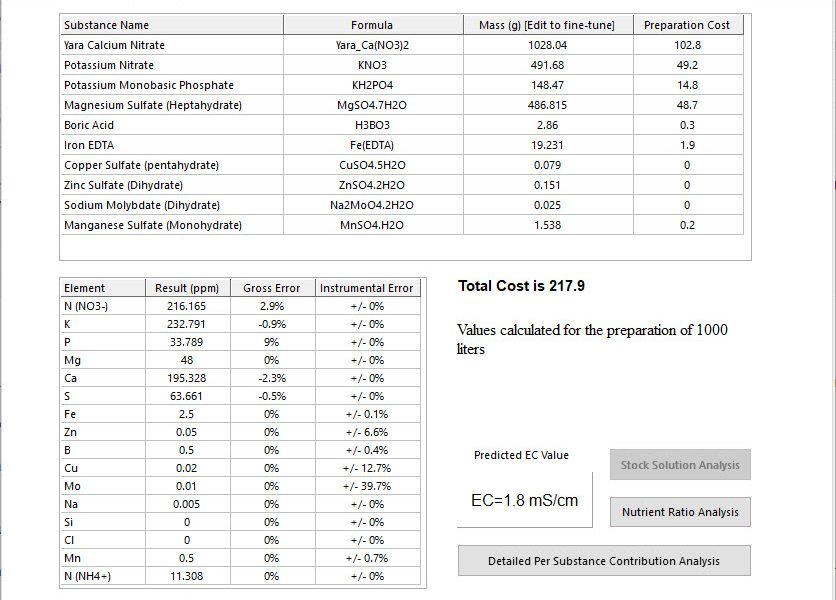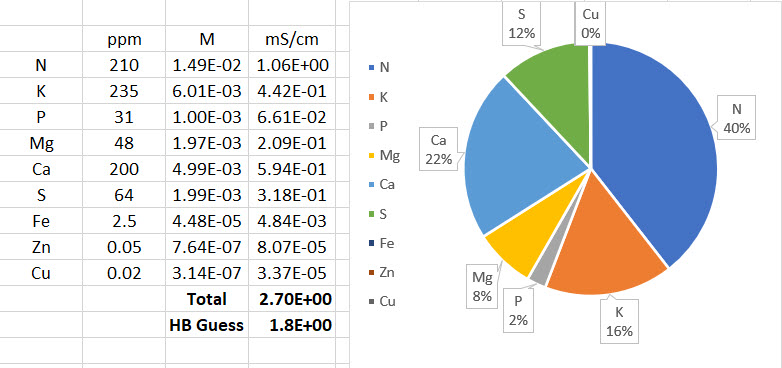# Nutrient solution conductivity estimates in HydrobuddyPeople who use Hydrobuddy can be confused by its conductivity estimates, especially because its values can often mismatch the readings of conductivity meters in real life. This confusion can stem from a lack of understanding of how these values are calculated and the approximations and assumptions that are made in the process. In this post I want to talk about theoretically calculating conductivity, what the meters read and why Hydrobuddy’s estimations can deviate from actual measurements.

The images above show the use of HydroBuddy for the calculation of a standard Hoagland solution for a 1000L reservoir. The Hoagland solution’s recipe is expressed as a series of elemental concentrations, all of them in parts per million (ppm) units. The results show that the final conductivity of this solution should be 1.8 mS/cm but in reality the conductivity of a freshly prepared full strength Hoagland solution will be closed to 2.5mS/cm. You will notice that HydroBuddy failed to properly calculate this value by an important margin, missing the mark by almost 30%. But how does HydroBuddy calculate this value in the first place?

Conductivity cannot be calculated by using the amount of dissolved solids in terms of mass because charges are transported per ion and not per gram of substance. To perform a conductivity calculation we first need to convert our elemental values to molar quantities and then associate these values with the limiting molar conductivity of each ion, because each ion can transport charge differently (you can find the values HydroBuddy uses in the table available in this article). This basically means we’re finding out how many ions we have of each kind and multiplying that amount by the amount each ion can usually transport if it were by itself in solution. The sum is the first estimate in the calculation of conductivity.Conductivity calculations carried out by HydroBuddy, also showing conductivity contributions per ion. This is done by converting ppm quantities to moles, then multiplying by limiting molar conductivity values here.

The image above shows the result of these calculations for an example with a perfectly prepared Hoagland solution. You can see that the estimate from limiting molar conductivity is initially 2.7 ms/cm – much closer to the expected 2.5 mS/cm – but then HydroBuddy makes an additional adjustment that lowers this down to 1.8 mS/cm. This is done because limiting molar conductivity values make the assumption of infinite dilution – what the ion conducts if it were all by itself in solution – but in reality the presence of other ions can decrease the actual conductivity things have in solution. HydroBuddy accounts for this very bluntly, by multiplying the result by 0.66, in effect assuming that the measured value of conductivity will be 66% of the value calculated from the limiting molar conductivity values. This is of course wrong in many cases, because the reduction in activity due to the presence of other ions is not as strong. However it can also be correct in many cases, primarily depending on the substances that are used to prepare the formulations and the ratios between the different nutrients.

In my experience HydroBuddy tends to heavily underestimate the conductivity of solutions that receive most of their conductivity from nitrates, as this example, but it tends to do much better when there are large contributions from sulfate ions. When I first coded HydroBuddy all my experiments were being done with much more sulfate heavy solutions, so the correction parameter value I ended up using for the program ended up being a bad compromise for solutions that deviated significantly from this composition. With enough data it might be possible to come up with a more advanced solution to conductivity estimations in the future that can adjust for non-linear relationships in the conductivity and activity relationships of different ions in solution.

If your measured conductivity deviates from the conductivity calculated in HydroBuddy you should not worry about it, as HydroBuddy’s values is meant to be only a rough estimate to give you an idea of what the conductivity might be like but, because of its simplicity, cannot provide a more accurate value at the moment. The most important thing is to ensure that all the salts, weights and volumes were adequately measured in order to arrive at the desired solution.

• […] yet a very poorly understood one. I have written several posts about conductivity in the past (1,2,3) and today I want to talk about the use of the term “Total Dissolved Solids” and the […]

•chivas
June 11, 2020 @ 8:56 pm

Thank you for the clarification. Any chance the 66% can be updated to a higher number in the next version? Or maybe a setting that user can use to calibrate the modifier to match their EC measurement?

•June 12, 2020 @ 8:27 am

Thanks for your comment! I’m actually working on building a model that can predict EC accurately, this will replace the currently used approximations.

•Robert
October 16, 2020 @ 3:27 am

Dear Daniel, I’d like to say Thank You for your continous work on improving Hydrobuddy.

Subscribe Today!